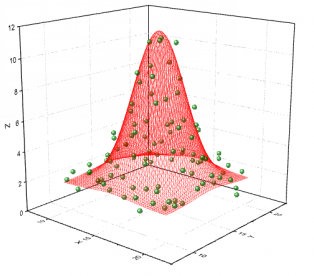# Problem in fitting (in function!)

Dear Rooters,

I was trying to fit the data from the below attached text file. The macro I was using is also attached (edit: now removed). I could not fit it. Any kind of help is appreciated.

data.txt (240.2 KB)

Dear @Wile_E_Coyote

Sorry about the parameter number. But, even after correcting for those two (as you mentioned), it does not fit. It gives error like:

``````Warning in <TCanvas::ResizePad>: Inf/NaN propagated to the pad. Check drawn objects.
Warning in <TCanvas::ResizePad>: Test width changed from 0 to 10
Error in <TPainter3dAlgorithms::FindLevelLines>: number of points for line not equal 2
``````

Any idea?

In your “fg” function, you blindly make calculations which may produce “±nan” and “±infinity” values.
You could try:

``````// if (TMath::IsNaN(valg) || TMath::IsNaN(-valg) || (valg == TMath::Infinity()) || (valg == -TMath::Infinity())) { std::cout << valg << std::endl; }
if (!((valg > -1000.) && (valg < 1000.))) valg = 0.; // a very brutal fix
return valg;
``````

That helps. Thank you very much.

Looking at your data, I guess you’d better try to use a TGraph2D, e.g.:

``````{
TGraph2D *g = new TGraph2D("data.txt");
g->SetTitle("some Thing;some X;some Y;some Z");
g->Draw("surf3"); g->Draw("pcol same");
#if 0 /* 0 or 1 */
g->GetXaxis()->SetLimits(50., 200.);
g->GetYaxis()->SetLimits(80., 280.);
#else /* 0 or 1 */
g->GetXaxis()->SetRangeUser(50., 200.);
g->GetYaxis()->SetRangeUser(80., 280.);
#endif /* 0 or 1 */
}
``````

@couet There is a bug in the drawing routines. One cannot use TAxis::SetLimits, if one wants “surf3” and “pcol” together. However, TAxis::SetRangeUser does work in this case.

Also, it may help if you use:
`g->Fit(fit1, "R"); // use the Range specified in the function range`

Dear @Wile_E_Coyote

Thanks. But, the macro you have shown regarding TGraph2D, is it plotting my data? I tried but in vain.

I modified the macro so that you can now explicitly see the data points, too.

The limits I see on the X and Y axis seems those set by SetLimits.

@couet The data points drawn by “pcol” are shifted with respect to the “surf3” shapes (if one uses TAxis::SetLimits).

but, somehow I am unable to plot it using your modified macro. I am using 5.34.36

Sorry, it seems that ROOT 5 has real problems with `g->Draw("surf3");` (maybe because this graph has 150x175 points on a “regular rectangular grid”). Maybe @couet knows any trick to get it working with ROOT 5 (not even “colz” works well but I think it begins with the problem that `g->GetHistogram()` takes ages to complete so I suspect that it’s a “known problem” with the Delaunay triangles).

@Sandy Can you possibly move to ROOT 6.20/04?
If you cannot, I’m afraid that you may need to stay with a TH2, e.g.:

``````{
// "data.txt" contains a 150x175 "regular rectangular grid" (26250 points)
TH2D *h = new TH2D("h", "some Thing;some X;some Y;some Z",
150, 0., 300., 175, 0., 350.);
TGraph2D *g = new TGraph2D("data.txt");
h->FillN(g->GetN(), g->GetX(), g->GetY(), g->GetZ());
delete g; // no longer needed
h->Sumw2(kFALSE); // make sure "bin_error = sqrt(bin_content)"
h->ResetStats(); // reset the statistics including the number of entries
h->GetXaxis()->SetRangeUser(50., 200.);
h->GetYaxis()->SetRangeUser(80., 280.);
gStyle->SetOptStat("neMRiuo"); // underflows and overflows respect ranges
h->Draw("surf3");
}
``````

@couet There is also another problem that I found. According to the “How to set ranges on axis?”, one should be able to use TAxis::SetLimits BEFORE the graph is drawn. This does not work here at all. I needed the following (note: no “pcol” at all so it’s another problem):

``````g->Draw("surf3"); // it MUST be drawn first
g->GetXaxis()->SetLimits(50., 200.); // now one can change it
``````

BTW. TAxis::SetRangeUser also needed canvas “Update” before it could be used (which is not mentioned in the “How to set ranges on axis?” at all).

Dear @Wile_E_Coyote

I could plot before also and now with your way too. But, I have query about fit. Let me try to make you understand about my query with an already set example in root website.

@couet Is there any way to make the both fits transparent in the sample plot (“From f2”) given under " Drawing a sub-range of a 2D histogram; the [cutg] option" in “https://root.cern/doc/v612/classTHistPainter.html” ?

@couet Like the attached figure…is there any way to change palette in such a way that one of the abode two fits would look like this and another fit would be of another colour but again transparentDear @couet,

Any idea on plotting TH2 drawn with option E and the fit surface drawn
in the same canvas but having transparent color (shown in the figure
attached before).

You can try to play with this:

``````{
TGraph2D *g = new TGraph2D("data.txt");
g->SetTitle("some Thing;some X;some Y;some Z");
#if 1 /* 0 or 1 */
Double_t zmin = 1.; // e.g. -1. or 0. or 1.
for (int i = (g->GetN() - 1); i >= 0; i--)
if (g->GetZ()[i] <= zmin) g->RemovePoint(i); // remove if "z" <= "zmin"
#endif /* 0 or 1 */
g->SetMarkerStyle(kCircle); g->SetMarkerSize(0.5);
g->Draw("pcol fb");
// g->Draw("surf3 fb"); g->Draw("pcol fb same");
#if 0 /* 0 or 1 */
g->GetXaxis()->SetLimits(50., 200.);
g->GetYaxis()->SetLimits(80., 280.);
#else /* 0 or 1 */
g->GetXaxis()->SetRangeUser(50., 200.);
g->GetYaxis()->SetRangeUser(80., 280.);
#endif /* 0 or 1 */
}
``````

Then you can use:

``````g->Fit(fit1, "R");
fit1->Draw("surf fb"); g->Draw("pcol fb same");
``````

@couet And there’s another bug … the markers are shifted with respect to the function if one uses (due to differences in axes ranges between the graph and the function?):

``````g->Fit(fit1, "R");
g->Draw("pcol fb"); fit1->Draw("surf fb same");
``````

Dear @Wile_E_Coyote,
That’s a nice one.

@couet But, how can I make the fit surface of monocolour, and transparent, no net like structure?

Note that you can also play with:
`fit1->SetLineColor(...); fit1->SetLineWidth(...); fit1->SetLineStyle(...);`

Sorry to come back on that only but the last days were holidays here.
Can you help me and tell me what I should look at now ?

From my side … there are three bugs reported in my previous posts here:

From @Sandy … I guess she wants to get a transparent color plot